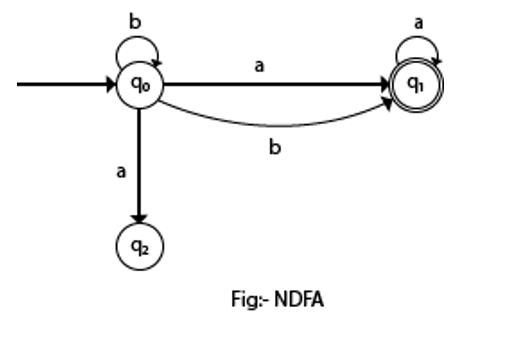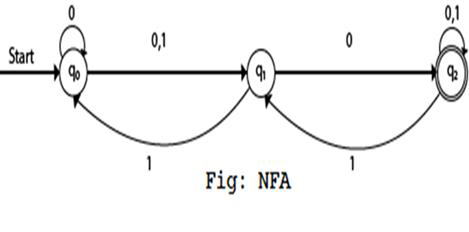# Explain Non-Deterministic Finite Automata in TOC.

NFA stands for non-deterministic finite automata. It is easy to construct an NFA when compared to DFA for a given regular language.

The finite automata are called NFA when there exist many paths for specific input from the current state to the next state.

Each NFA can be translated into DFA but every NFA is Non DFA.

NFA is defined in the same way as DFA but with the following two exceptions, which are as follows −

• It contains multiple next states.
• It contains ε transitions.

## Example

The transition diagram is as follows −In the above NFA, it is clear that there exist many paths for specific input from the current state to the next state. (state q0 on input ‘b’ it goes to q0 itself and q1).

Non-deterministic finite automata also have five states which are same as DFA, but with different transition function, as shown follows −

δ: Q X Σ -> 2Q

Non-deterministic finite automata is defined as a 5 tuple,

M=(Q, Σ, δ,q0,F)

Where,

• Q: Finite set of states
• Σ: Finite set of the input symbol
• q0: Initial state
• F: Final state
• δ: Transition function: Q X Σ -> 2Q

## Graphical Representation of an NFA

An NFA can be represented by digraphs called state diagrams.

The following factors are considered while graphically representing NFA −

• The state is represented by vertices.
• The arc labelled with an input character shows the transitions.
• The initial state is marked with an arrow.
• The final state is denoted by the double circle.

Q = {q0, q1, q2}

Σ = {0, 1}

q0 = {q0}

F = {q2}

## Transition diagram

The transition diagram is as follows −## Transition table

The transition table is as follows −

Present stateNext state for input 0Next state for input
->q0q0,q1q1
q1q2q0
*q2q2q1,q2

## Explanation

• Step 1 − q1 is an initial state on input ‘0’ it goes to multiple states q0 and q1, on input ‘1’ goes to state q1.
• step 2 − q1 is an intermediate state on input ‘0’ goes to state q2 which is a final state and on input ‘1’ goes to state q0.
• Step 3 − q2 is the final state on input ‘0’ goes to q2 itself and on ‘1’ it goes to multiple states q1 and q2.